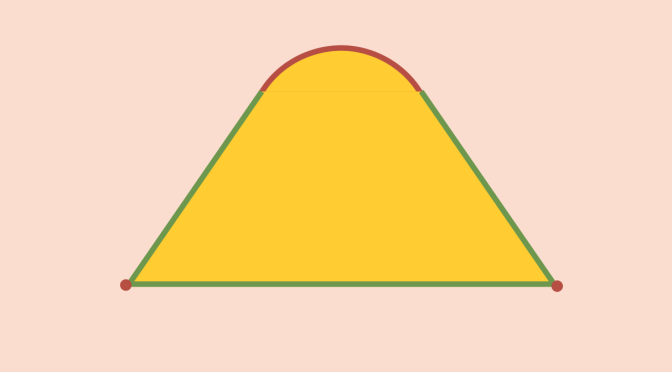A counterexample to Krein-Milman theorem

In the theory of functional analysis, the Krein-Milman theorem states that for a separated locally convex topological vector space $$X$$, a compact convex subset $$K$$ is the closed convex hull of its extreme points.

For the reminder, an extreme point of a convex set $$S$$ is a point in $$S$$ which does not lie in any open line segment joining two points of S. A point $$p \in S$$ is an extreme point of $$S$$ if and only if $$S \setminus \{p\}$$ is still convex.

In particular, according to the Krein-Milman theorem, a non-empty compact convex set has a non-empty set of extreme points. Let see what happens if we weaken some hypothesis of Krein-Milman theorem.

The closed unit ball of $$L^1([0,1])$$ has no extreme points

Consider the vector space $$L^1([0,1])$$ of all equivalent classes of Lebesgue integrable functions defined on $$[0,1]$$. $$L^1([0,1])$$ is a Banach space equipped with the norm $\Vert f \Vert_1 = \int_0^1 \vert f(t) \vert dt$ Hence $$L^1([0,1])$$ is a separated locally convex topological vector space.

We prove that the closed unit ball $$B$$ of $$L^1([0,1])$$ has no extreme points. First, it is easy to prove that $$f \in B$$ with $$\Vert f \Vert_1 < 1$$ cannot be an extreme point. Then consider $$f \in L^1([0,1])$$ with $\Vert f \Vert_1=\int_0^1 \vert f(t) \vert dt = 1$ One can find $$c \in (0,1)$$ such that $\int_0^c \vert f(t) \vert dt = \int_c^1 \vert f(t) \vert dt = \frac{1}{2}$ Let $f_1(x) = \begin{cases} 2 f(x) & \mbox{if } x \in [0,c) \\ 0 & \mbox{if } x \in [c,1] \end{cases}$ and $f_2(x) = \begin{cases} 0 & \mbox{if } x \in [0,c) \\ 2 f(x) & \mbox{if } x \in [c,1] \end{cases}$ Then $$f$$ is the middle point of the segment $$[f_1,f_2]$$ whose end points both belong to $$B$$.